## Thursday, February 02, 2006

### Exactly chiral fermions

In the last post in this series, we looked at the Ginsparg-Wilson relation and how it might provide a way to get past the Nielsen-Ninomiya theorem. In this post we shall have a look at how this can happen in practice.

One way in which a four-dimensional theory of a chiral fermion can be realized is by dimensional reduction from a five-dimensional theory. Let us consider the five-dimensional continuum theory of a Dirac fermion coupled to a scalar background field depending on only the fifth dimension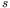: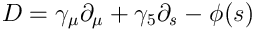where the scalar field is assumed to be a step function of the same general form as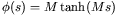. The plane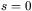can be understood as a domain wall of width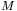separating domains of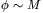and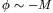. The case of interest haslarge.

From the square of the Dirac equation, we have for a fermion with four-momentum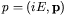,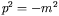,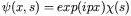, that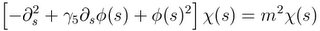and the allowed masses on the four-dimensional domain wall are determined by the eigenvalue spectrum of a differential operator in. All non-zero eigenvalues are of orderand hence large. For the zero eigenvalues, the Dirac equation can be decoupled into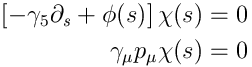with solutions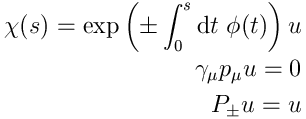Of these, only the negative chirality solution is normalizable, and hence the low-energy spectrum on the domain wall consists of a single left-handed chiral fermion.

The presence of the scalar background field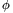is a little awkward, but we may simplify the situation to the case of an ultra-massive five-dimensional fermion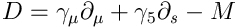in the half-space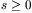subject to the Dirichlet boundary condition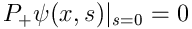and perform the same analysis withreplaced by.

In the early nineties, Kaplan discovered that the same domain wall effect still occured on a lattice when the Wilson operator was used to discretize the five-dimensional theory. The apparent violation of the Nielsen-Ninomiya theorem is due to the fact that the four-dimensional theory is not the whole story: with a finite extent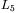in the fifth direction, there will necessarily be another domain wall with opposite orientation, on which a massless chiral fermion of opposite chirality will live, thus fulfilling both the Nielsen-Ninomiya theorem in the five-dimensional theory and ensuring the mutual cancellation of the chiral anomalies stemming from either fermion. The anomalous divergence simply becomes a flow of charge onto and off the domain wall from the extra dimension.

Around the same time, Narayanan and Neuberger discovered a formulation of chiral fermions in terms of the overlap between the ground states of two Hamiltonians representing "time" evolution to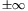along the fifth direction. Later, Neuberger discovered a way to write the overlap as the determinant of a Dirac operator, the overlap operator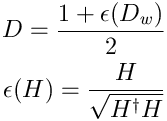where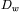is the Wilson Dirac operator. This formulation avoids the need for an explicit fifth dimension, but at the expense of introducing the slightly awkward operator sign function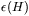.

Later, it was shown that the domain wall and overlap formulations were essentially equivalent. It can also be shown that both the overlap operator and the effective Dirac operator for fermions on the domain wall satisfy the Ginsparg-Wilson relation, thereby allowing to describe exactly chiral fermions on the lattice.

So what is the bad news? The bad news is that these exactly chiral fermion formulations are extremely hard to simulate. Domain wall fermions need to be simulated in five dimensions, greatly increasing the compuational demand, and for overlap fermions the operator sign function is rather difficult to compute. So while these actions are exactly chiral, and hence in way closer to the real continuum physics, simulating them at reasonable sizes and lattice spacing will require a huge computational effort. If one considers to what effort MILC had to go to get 1% level predictions using staggered fermions (which are very efficient to simulate), it becomes clear that high-precision predictions from dynamical simulations using exactly chiral fermions are still a fair while in the future.

In the next, and probably final post in this series, we will go and have a look at a fairly new lattice fermion action, known as twisted mass.